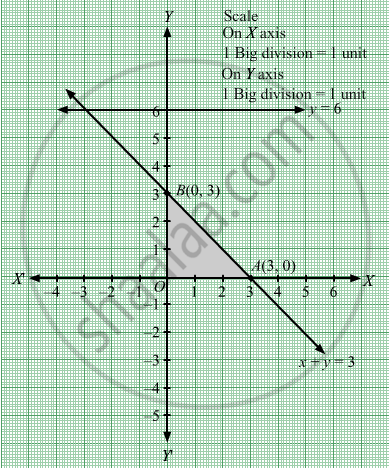Share
Notifications

View all notifications
Books Shortlist
Your shortlist is empty

# The Region Represented by the Inequation System X, Y ≥ 0, Y ≤ 6, X + Y ≤ 3 is (A) Unbounded in First Quadrant (B)Unbounded in First and Second Quadrants (C) Bounded in First Quadrant (D) None of These - CBSE (Commerce) Class 12 - Mathematics

Login
Create free account

Forgot password?
ConceptGraphical Method of Solving Linear Programming Problems

#### Question

The region represented by the inequation system xy ≥ 0, y ≤ 6, x + y ≤ 3 is

• unbounded in first quadrant

• unbounded in first and second quadrants

•  bounded in first quadrant

•  none of these

#### Solution

bounded in first quadrant
Converting the given inequations into equations, we obtain
$y = 6, x + y = 3, x = 0 \text{ and }y = 0$ y = 6 is the line passing through (0, 6) and parallel to the X axis.The region below the line y = 6 will satisfy the given inequation.

The line x + y = 3 meets the coordinate axis at A(3, 0) and B(0, 3). Join these points to obtain the line x + y =3.
Clearly, (0, 0) satisfies the inequation x + y ≤ 3. So, the region in xy-plane that contains the origin represents the solution set of the given equation.

Region represented by x ≥ 0 and y ≥ 0:
Since, every point in the first quadrant satisfies these inequations. So, the first quadrant is the region represented by the inequations.
These lines are drawn using a suitable scale.The shaded region represents the feasible region of the given LPP, which is bounded in the first quadrant

Is there an error in this question or solution?

#### Video TutorialsVIEW ALL 

Solution The Region Represented by the Inequation System X, Y ≥ 0, Y ≤ 6, X + Y ≤ 3 is (A) Unbounded in First Quadrant (B)Unbounded in First and Second Quadrants (C) Bounded in First Quadrant (D) None of These Concept: Graphical Method of Solving Linear Programming Problems.
S